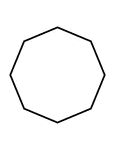### Flashcard of a polygon with eight equal sides

A flashcard featuring an illustration of a polygon with eight equal sides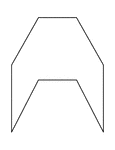### Irregular Concave Octagon

Illustration of an irregular concave octagon. This polygon has some symmetry.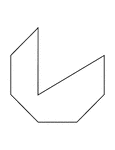### Irregular Concave Octagon

Illustration of an irregular concave octagon.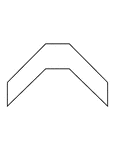### Irregular Concave Octagon

Illustration of an irregular concave octagon. This polygon has some symmetry.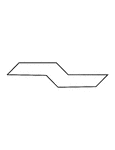### Irregular Concave Octagon

Illustration of an irregular concave octagon. This polygon has some symmetry.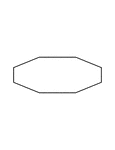### Irregular Convex Octagon

Illustration of an irregular convex octagon. This polygon has some symmetry.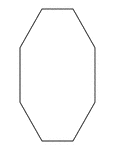### Irregular Convex Octagon

Illustration of an irregular convex octagon. This polygon has some symmetry.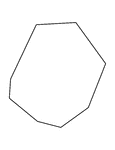### Irregular Convex Octagon

Illustration of an irregular convex octagon.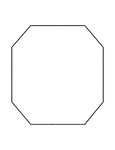### Irregular Convex Octagon

Illustration of an irregular convex octagon. This polygon has some symmetry.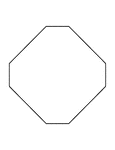### Irregular Convex Octagon

Illustration of an irregular convex octagon. This polygon has some symmetry.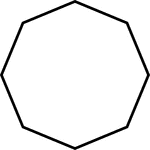### 8-sided Polygon

Polygon consisting of 8 sides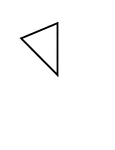### Fractions of 8-sided Polygon

1/8 of a 8 sided polygon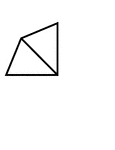### Fractions of 8-sided Polygon

2/8 of a 8 sided polygon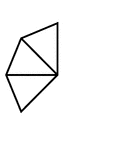### Fractions of 8-sided Polygon

3/8 of a 8 sided polygon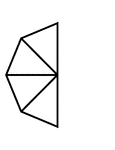### Fractions of 8-sided Polygon

4/8 of a 8 sided polygon### Fractions of 8-sided Polygon

5/8 of a 8 sided polygon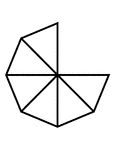### Fractions of 8-sided Polygon

6/8 of a 8 sided polygon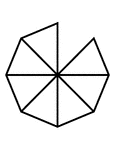### Fractions of 8-sided Polygon

7/8 of a 8 sided polygon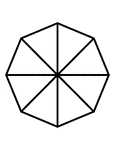### Fractions of 8-sided Polygon

8/8 of a 8 sided polygon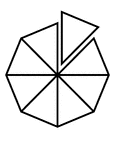### Fractions of 8-sided Polygon

8/8 of a 8 sided polygon with one piece detached